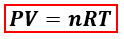# Problem: Calculate the volume occupied by 35.2 g of methane gas (CH4) at 25 °C and 1.0 atm. R = 0.08206 L•atm/mol•Ka) 0.0186 Lb) 4.5 Lc) 11.2 Ld) 48.2 Le) 53.7 L

🤓 Based on our data, we think this question is relevant for Professor N/A's class at Ryerson University.

###### FREE Expert Solution

We are asked to find the volume of methane gas (CH4) at the given conditions. Since mass, temperature, and pressure are given, we will use the ideal gas equation to calculate for the volume.P = pressure, atm
V = volume, L
n = moles, mol
R = gas constant = 0.08206 (L·atm)/(mol·K)
T = temperature, K

In the ideal gas equation, moles are needed but the mass of CH4 is given instead. We’ll calculate the moles of CH4 using its mass and molar mass.###### Problem Details

Calculate the volume occupied by 35.2 g of methane gas (CH4) at 25 °C and 1.0 atm. R = 0.08206 L•atm/mol•K

a) 0.0186 L

b) 4.5 L

c) 11.2 L

d) 48.2 L

e) 53.7 L

What scientific concept do you need to know in order to solve this problem?

Our tutors have indicated that to solve this problem you will need to apply the The Ideal Gas Law concept. You can view video lessons to learn The Ideal Gas Law. Or if you need more The Ideal Gas Law practice, you can also practice The Ideal Gas Law practice problems.

What is the difficulty of this problem?

Our tutors rated the difficulty ofCalculate the volume occupied by 35.2 g of methane gas (CH4)...as low difficulty.

How long does this problem take to solve?

Our expert Chemistry tutor, Sabrina took 3 minutes and 51 seconds to solve this problem. You can follow their steps in the video explanation above.

What professor is this problem relevant for?

Based on our data, we think this problem is relevant for Professor N/A's class at Ryerson University.